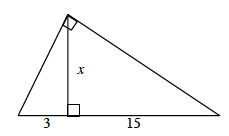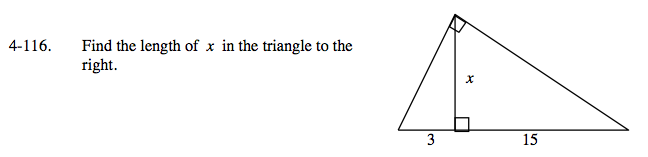### Home > PC > Chapter 4 > Lesson 4.2.4 > Problem4-116

4-116.

Find the length of x in the triangle below. Homework Help ✎These are similar triangles. Set up a proportion and solve for x.

$\frac{3}{x}=\frac{x}{15}$